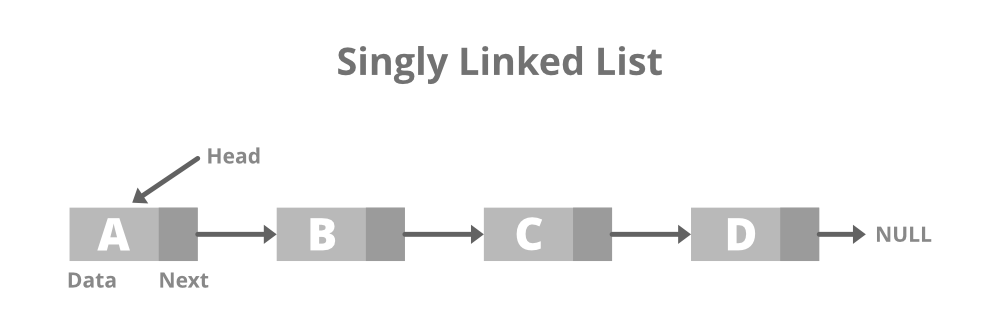×

Search anything:

# Implementing Linked list in Python using OOP conceptsGet this book -> Problems on Array: For Interviews and Competitive Programming

## What is a Linked List?

A Linked List is a data structure where each element holds 2 pieces of information: it's value and the reference to the next element. A reference is like a pointer or a clue that tells where to find something.

Imagine that there are many boxes. Each box has something inside it. These boxes are connected to each other with arrows such that each box knows where the next box is. A Linked List is similar to this arrangement of boxes. In a linked list, each box, called a node, contains a value and the location of the next box or node.

To find an item in a linked list, we start at the first box (node), look inside the box. Then we follow the arrow to the next box (node) and repeat this till we find the item we're looking for.Linked Lists are different from Arrays in the sense that they dont require fixed space. We can add and delete elements easily. Also, in arrays, elements are stored in a contiguous way whereas in linked lists it is not the case.

Now, let's understand how to implement a linked list in Python using OOP concepts.

## Node Class

We will first create a class for nodes to represent each node in the linked list. Each node will have 2 properties, it's value and the reference of the next node.

1. __init__ initializes a new node with the given value. next is originally set as None which implies that the node doesn't point to any other node initially.
2. get_value(self) returns the value stored in the node, allowing access to the value from outside the class.
3. get_next(self) retrieves the reference to the next node in the linked list.
4. set_next(self, next_node) sets the reference to the next node. It links the current node to the specified next node.
``````class Node:
def __init__(self, val):
self.val = val
self.next = None

def get_value(self):
return self.val

def get_next(self):
return self.next

def set_next(self, next_node):
self.next = next_node
``````

Next, we will create a class for Linked List itself. In the class we will add basic methods for insert, delete, print and search.

__init__ initializes Linked List with head, which points to the first node of the linked list. It is set as None because initially, the linked list is empty.

``````class LinkedList:
def __init__(self):
``````

We can now add some basic methods to modify the linked list.

In this method, we create a new node with the given value and then set the next attribute of this new node to the current head node. Finally, we update head to point to this new node.

`````` def add_at_beginning(self, val):
new_node = Node(val)
``````

In this method, we create a new node with the given value and then set the next attribute of the last node to the new node.

`````` def add_at_end(self, val):
new_node = Node(val)
else:
while curr_node.next:
curr_node = curr_node.next
curr_node.next = new_node
``````

### Delete method

In this method, we delete the node with the given value. First, we check if the linked list is empty. Then we check if the node to be deleted is the head of the linked list. Finally, we iterate over the list to find the value which if found is deleted.

`````` def delete(self, val):
return

return

prev_node = None
while curr_node and curr_node.get_value() != val:
prev_node = curr_node
curr_node = curr_node.get_next()

if curr_node:
prev_node.set_next(curr_node.get_next())
``````

### Printing elements of the linked list

In this method, we print the elements of the linked list one by one by iterating over it.

``````def print(self):
while curr_node:
print(curr_node.val,end = " --> ")
curr_node = curr_node.next
print("None")
print("\n")
``````

### Searching a value

In this method, we will implement a basic search operation for a given value. We will go through the list till we find the value and return True. Otherwise we will return False if not found.

``````def search(self, val):
while curr_node:
if curr_node.get_value() == val:
return True
curr_node = curr_node.get_next()
return False
``````

### Putting it all together

Now that we have all the methods, we will bring them together and complete our Class definitions for nodes and linked lists.

``````class Node:
def __init__(self, val):
self.val = val
self.next = None

def get_value(self):
return self.val

def get_next(self):
return self.next

def set_next(self, next_node):
self.next = next_node

def __init__(self):

new_node = Node(val)

new_node = Node(val)
else:
while curr_node.next:
curr_node = curr_node.next
curr_node.next = new_node

def delete(self, val):
return

return

prev_node = None
while curr_node and curr_node.get_value() != val:
prev_node = curr_node
curr_node = curr_node.get_next()

if curr_node:
prev_node.set_next(curr_node.get_next())

def print(self):
while curr_node:
print(curr_node.val,end = " --> ")
curr_node = curr_node.next
print("None")
print("\n")

def search(self, val):
while curr_node:
if curr_node.get_value() == val:
return True
curr_node = curr_node.get_next()
return False
``````

Now that we have our complete code, let's see one example to see how to create a linked list and perform basic operations on it in Python

## Example

``````list = LinkedList()
list.print()

list.print()

list.delete(3)
list.print()

print(list.search(3))
print(list.search(4))
``````

The following will be the output

``````1 --> 2 --> 3 --> None
1 --> 2 --> 3 --> 4 --> None
1 --> 2 --> 4 --> None
False
True
``````

To conclude, in this OpenGenus article we implemented a linked list in Python using OOP concepts. We defined a Node class to represent each node in the linked list and then we defined a LinkedList class to manage the linked list, providing methods to add, delete, search and print elements. You can create your own methods and perform various operations on the linked list using these various methods.

#### Aayush Shah

I am an enthusiastic Engineer from Birla Institute of Technology and Science, Pilani, driven by a newfound passion for open source contribution and the belief in freely accessible resources for all.